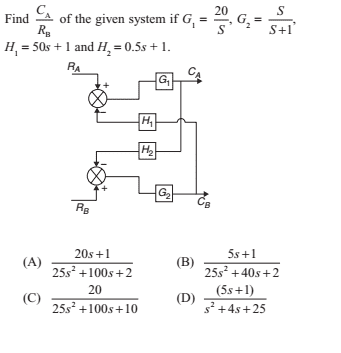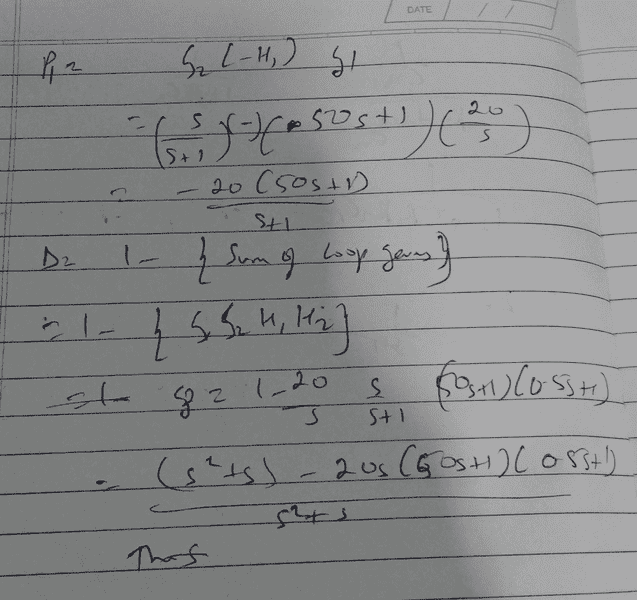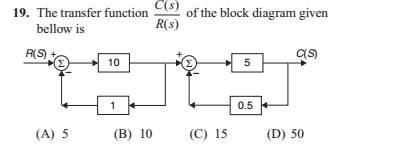# Control System: Find the Transfer Function From Block Diagram

jaus tail

## Homework Statement## Homework Equations

To find Ca / Rb we take Ra = 0 --- is this right?
I used mason's formula
P1 = -G2 G1 H1
Loop = G1, G2, -H1, -H2
Using Mason's Gain we get:
Delta1 = 1
Delta = 1 - G1G2H1H2
Using Mason's Gain
TF = -G1G2H1 Divided by ( 1 - G1G2H1H2)
But substituting the values I don't get the answer that matches any options.

## The Attempt at a Solution

Substituting I get:This gives answer in cube as second term of numerator will go in cube for s. None of options have s cube.

Second Question:I think the options are wrong.
For left TF we get C(s)/R(s) = 10/11
For Right we get C(s)/R(s) = 5/3.5
Multiplying them doesn't give the answer

#### Attachments

Homework Helper
Gold Member
It's been awhile since I've done these, but I got the same result as you, for the second one: C/R = 100/77. I haven't worked through the first one, yet.

Gold Member
To find Ca / Rb we take Ra = 0 --- is this right?
yes
I got the same thing as you for the second problem.

In problem number one you can dive both the top and bottom side by s...

•jaus tail
jaus tail
Not sure if that helps.
I'm getting: Ca/Rb = -G2 H1 G1 divided by (1 + ( -G2 H1 G1 H2 ) )
And that's not reducing to any of the options.

Sounds like the problem might be "hosed"•